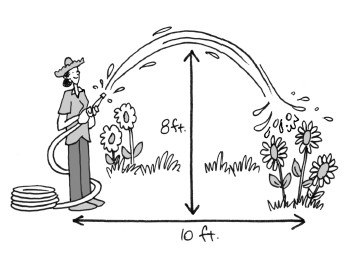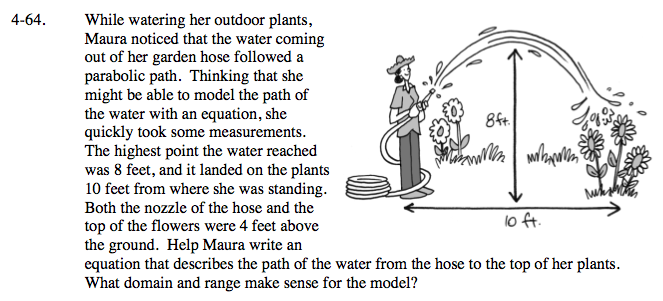### Home > A2C > Chapter Ch4 > Lesson 4.2.1 > Problem4-64

4-64.While watering her outdoor plants, Maura noticed that the water coming out of her garden hose followed a parabolic path. Thinking that she might be able to model the path of the water with an equation, she quickly took some measurements. The highest point the water reached was 8 feet, and it landed on the plants 10 feet from where she was standing. Both the nozzle of the hose and the top of the flowers were 4 feet above the ground. Help Maura write an equation that describes the path of the water from the hose to the top of her plants. What domain and range make sense for the model? 4-64 HW eTool (Desmos). Homework Help ✎There are several ways to write the equation. It depends on how you decide to draw the x- and y-axes.

The y-axis could go through the point where the water leaves the hose at (0, 4). On the other hand you could put the y-axis through the vertex and place the x-axis so that the nozzle is at (−4, 0). Still another possibility is to make the vertex the point (0, 0).

Use the general equation of a parabola in graphing form: y = a(xh)2 + k

After you've filled in the values for h and k, you will need to substitute one point (x, y) in order to solve for a. Then you will be able to write the equation.

Use the eTool below to check your work.
Click the link at right for the full version of the eTool: A2C 4-64 HW eTool